Hydrol. Earth Syst. Sci., 25, 5917–5935, 2021
https://doi.org/10.5194/hess-25-5917-2021
Hydrol. Earth Syst. Sci., 25, 5917–5935, 2021
https://doi.org/10.5194/hess-25-5917-2021

Research article 15 Nov 2021

Research article | 15 Nov 2021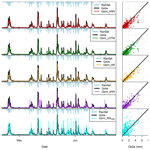# Evaluating different machine learning methods to simulate runoff from extensive green roofs

Evaluating different machine learning methods to simulate runoff from extensive green roofs
Elhadi Mohsen Hassan Abdalla1, Vincent Pons1, Virginia Stovin2, Simon De-Ville2, Elizabeth Fassman-Beck3, Knut Alfredsen1, and Tone Merete Muthanna1 Elhadi Mohsen Hassan Abdalla et al.
• 1Department of Civil and Environmental Engineering, Norwegian University of Science and Technology, Trondheim, 7031, Norway
• 2Department of Civil and Structural Engineering, The University of Sheffield, Sheffield, S1 3JD, UK
• 3Southern California Coastal Water Research Project, Costa Mesa, CA 92626, USA

Abstract

Green roofs are increasingly popular measures to permanently reduce or delay storm-water runoff. The main objective of the study was to examine the potential of using machine learning (ML) to simulate runoff from green roofs to estimate their hydrological performance. Four machine learning methods, artificial neural network (ANN), M5 model tree, long short-term memory (LSTM) and k nearest neighbour (kNN), were applied to simulate storm-water runoff from 16 extensive green roofs located in four Norwegian cities across different climatic zones. The potential of these ML methods for estimating green roof retention was assessed by comparing their simulations with a proven conceptual retention model. Furthermore, the transferability of ML models between the different green roofs in the study was tested to investigate the potential of using ML models as a tool for planning and design purposes. The ML models yielded low volumetric errors that were comparable with the conceptual retention models, which indicates good performance in estimating annual retention. The ML models yielded satisfactory modelling results (NSE >0.5) in most of the roofs, which indicates an ability to estimate green roof detention. The variations in ML models' performance between the cities was larger than between the different configurations, which was attributed to the different climatic characteristics between the four cities. Transferred ML models between cities with similar rainfall events characteristics (Bergen–Sandnes, Trondheim–Oslo) could yield satisfactory modelling performance (Nash–Sutcliffe efficiency NSE >0.5 and percentage bias |PBIAS|<25 %) in most cases. However, we recommend the use of the conceptual retention model over the transferred ML models, to estimate the retention of new green roofs, as it gives more accurate volume estimates. Follow-up studies are needed to explore the potential of ML models in estimating detention from higher temporal resolution datasets.

Share
1 Introduction

Green roofs are a type of green infrastructure (GI) that have received significant attention in recent years. In contrast to conventional storm-water infrastructure, green roofs attempt to decrease storm-water outflows while providing other services, such as reducing urban heat island effect, preserving the cities ecosystems and improving the urban visual amenity among other benefits . Roof areas represent around 40 %–50 % of impermeable areas in dense urban catchments ; therefore, retrofitting current roofs with substrate/growing media and vegetation offers an efficient and area-free GI option. Many studies have confirmed the potential of green roofs to mitigate rainfall events from field measurements .

Quantifying the hydrological performance of a green roof is usually done by estimating “retention”, a permanent reduction of storm water by evapotranspiration, and “detention”, flow peak reduction and delay. Both retention and detention metrics are needed to justify the widespread implementation of green roofs by the storm-water community and for planning and design by practicing engineers. Hence, numerous studies have investigated different approaches and tools to simulate outflows from green roofs to estimate retention and detention metrics.

For estimating green roof detention, models that simulate rainfall–runoff events in short time steps (sub-hourly) are required. Several models have been tested successfully in the literature, which can be categorized into physically based and conceptual models. Physically based models simulate the water flow in porous media by solving physical equations numerically, such as the Richards equations, either in 1D , 2D or 3D . Several tools exist that can be used to implement this type of model, such as HYDRUS , SWMS-2D and COMSOL Multiphysics . These models have proven to be accurate and to rely only on measurable parameters and can be powerful tools for studies that aim at in-depth understanding of the hydraulic behaviours of the different green roof layers .

Another category of physically based models apply simplified and analytical forms of physical equations, such as the Green–Ampt equation for infiltration and the Darcy law for saturated water flow . Popular modelling tools that implement these models include the EPA-SWMM and MIKE URBAN (DHI2017). This category of models is perhaps the most commonly applied in the literature of green roof modelling, and it has been acknowledged by many studies to be a suitable tool for analysing the hydrological performance of green roofs . However, due to the simplicity of these models, they often rely on calibrated rather than measured parameters. found the simulated hydrographs of uncalibrated SWMM models to deviate significantly form the observed ones. attempted to transfer calibrated SWMM model parameters between similar green roofs located in different locations. However, only parameter sets from wet locations yielded good results in drier locations but not vice versa

Conceptual models simplify the physical processes using linear or nonlinear equations to simulate green roof runoff. One common type of these models is the reservoir routing model which was applied to estimate runoff detention from event-based simulations in previous literature . These models were found to produce results that are comparable to physically based models with lower level of complexity (i.e. reduced number of model parameters) . recommended the use of a reservoir routing model instead of physically based models for design purposes when little information is available about the green roof properties. However, the parameters of conceptual models are not measurable. Hence, calibration is needed to find their optimal values, unlike physically based models . A few studies have identified relations between the flow parameters of reservoir models and some physical properties of green roofs, such as slope and substrate depth . However, these studies focused on lab-scale green roofs in which detention due to the horizontal flow is not significant .

For estimating green roof retention, models with water balance equations (in hourly or daily time step) and suitable representation of the actual evapotranspiration process (AET) were found by many studies to be sufficient . The most common way to model AET is by multiplying the potential evapotranspiration (PET), the maximum evaporation rate assuming unlimited water supply, with reduction functions that account for soil moisture deficit and crop type. The reduction functions require careful parameterization of the maximum storage of the roof and crop factors. The maximum storage of the roof was found by many studies to be related to the measurable field capacity of the substrate . Crop factors for agricultural crops are well documented and studied . However, crop factor values for Sedum plants, commonly applied for green roofs, are less known. Previous studies reported different crop factor values for Sedum plants .

Data-driven models, which are derived entirely from observed data, may offer alternative modelling tools that can estimate both retention and detention of green roofs without explicitly accounting for complex hydrological processes. However, the use of data-driven models in green roof studies has been limited to simple regression models which are site-specific and not transferable. More advanced data-driven methods, such as machine learning (ML), have been commonly applied in many hydrological modelling studies in the last few decades. However, only a few studies were found to apply ML models in green infrastructures , and no study was found to apply ML models in estimating the hydrological performance of extensive green roofs.

Machine learning methods have been successfully applied in hydrological modelling in recent decades. Previous studies reported better performances of ML models compared to conventional hydrological models in runoff prediction , runoff simulation , and for building relationships between water level and discharge . Some of the popular machine learning methods applied in hydrology include artificial neural networks (ANNs), M5 model tree, long short-term memory (LSTM) and k nearest neighbour (kNN).

Artificial neural network is the most common and among the earliest ML used in hydrological modelling (Daniell1991). Early examples of research into ANN include the study conducted by , in which ANN outperformed the linear ARMAX and the conceptual Sacramento SAC-SMA model in simulating runoff from a medium-sized catchment. Likewise, compared an ANN to a simple conceptual model and found the former to outperform the latter. M5 model tree has been applied in different studies. reported a satisfactory performance of both M5 model tree and ANN in runoff forecasting. They, however, emphasized the advantages of M5 model tree over ANN due to the better interpretation of M5 model outputs. applied the M5 model tree for flow forecasting in India, among other ML methods, and found it to perform satisfactorily. Away from flow simulation, used M5 tree and other methods to simulate the spatial distribution of snow depths in Iran, while evaluated M5 model tree on formulating operation rules for a reservoir. Kisi (2016) used M5 model tree to model reference evapotranspiration.

LSTM has been applied in different scientific fields and could provide good results (Shen2018). Regarding runoff modelling, investigated the potential of LSTM to predict runoff from ungauged basins. They could achieve good prediction performance that was comparable to the well-known Sacramento model. Similarly, obtained comparable results with LSTM to a conceptual model. compared between an ANN and an LSTM in runoff simulation and found the latter to outperform the former. Nevertheless, LSTM is computationally expensive, and the training process takes a long time (Ayzel2019). The k nearest neighbour method was applied first by in runoff forecasting in which it outperformed unit hydrograph forecasters. found the k nearest neighbour to be comparable with ANN in monthly runoff forecasting. Furthermore, applied the k nearest neighbour in predicting monthly runoff, and they discussed the effect of k value on the performance of kNN.

Few studies have modelled green infrastructure with ML techniques. For instance, applied a Fuzzy-neural network to optimize irrigation of a green roof by estimating soil moisture deficit. The neural network could reproduce the soil moisture well, which indicates the capability of ML models to simulate the nonlinear AET process. developed an artificial neural network model to predict the flow reduction from a catchment with different GI structures. Similarly, applied a neural network model to predict flow reduction from a permeable pavement. The satisfactory performances of ML models in two studies demonstrate the potential of ML models in GI hydrological modelling.

This study examines the ability of four machine learning methods, M5 model tree, artificial neural networks (ANNs), long short-term memory (LSTM) and k nearest neighbour (kNN), to estimate green roof hydrological performance specifically by

1. evaluating the performance of ML models in simulating the temporal dynamics of green roof subsurface runoff and estimating the retention from long-term simulations across different climatic locations;

2. investigating the potential of using ML models as a useful tool for planning that predicts the performance of new green roofs when observations are not available.

2 Data

Sixteen extensive green roofs located in four Norwegian cities with different climates – Bergen (BERG), Sandnes (SAN), Oslo (OSL) and Trondheim (TRD) – were used in the study. Bergen city is located on the western coast of Norway. Bergen is the wettest city among the four with annual precipitation of 3110 mm followed by Sandnes city, which is located on the south-west coast, with annual precipitation of 1690 mm. Oslo is the driest city with only 970 mm of annual precipitation, while Trondheim is the northernmost city with annual precipitation of 1070 mm. According to the Köppen–Geiger climate classification , both Bergen and Sandnes are classified as temperate oceanic climate (Cfb), while Oslo has the warm-summer humid continental climate (Dfb), and Trondheim has a subpolar oceanic climate (Dfc). The locations of the four cities are shown in Fig. 1.

Table 1 shows the geometries and configurations of roofs. Roof geometries (areas and slopes) vary between the cities, while the different configurations represent the variety of options in the Norwegian green roof market. Some green roofs in the study have the same configuration, for instance, BERG1, SAN1 OSL3 and TRD1. Continuous time series data were collected from TRD, BERG and SAN roofs between 2015 to 2017, while the green roofs at OSL have a 7-year record of data from 2011 to 2017. Data includes precipitation, runoff, relative humidity and wind speed at a 1 min resolution. In Oslo, the wind speed was not measured at the roofs but collected from a nearby station. For details about roof setup, data collection and processing, please refer to .

Table 1Roof Geometries and Configurations.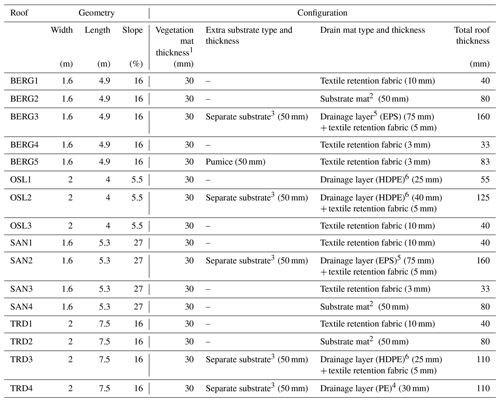1Pregrown reinforced vegetation mats (sedum).
2Substrate mat: a mineral wool plate.
3Separate substrate: a mixture of LECA and bricks.
4Drainage layer (PE): plastic drainage layers of polyethylene.
5Drainage layer (EPS): plastic drainage layers of expanded polystyrene.
6Drainage layer (HDPE): plastic drainage layers of high-density polyethylene.

3 Methods and tools

## 3.1 Machine learning models

### 3.1.1 M5 model tree

In this approach, the training data are divided into many subsets. For each subset, a piecewise linear regression equation is built between the output and the input variables . The algorithm used by the model tree is called M5, which was developed in 1992 . It divides the data into subsets based on rules that reduce the intra-variation (variance) within each subset (variables within each subset are as similar as possible). The M5 model tree has an upside-down tree structure. Input variables enter the tree from the top (the tree root) to arrive at the models located at the tree leaves. For a detailed explanation of the M5 model tree, see .

In this study, the Cubist library in R was used to build M5 models. The performance of Cubist-M5 models can be improved by tuning two hyperparameters, namely committees and neighbours. The former is the number of trees in a boost-like ensemble scheme where iterative M5 model trees are built in sequence. The first M5 tree is built following the M5 algorithm, while the subsequent trees are created from the residuals of the single tree. The final model prediction is the average from all M5 trees in the ensemble. The final prediction of a single tree can be improved by a post-model nearest-neighbour adjustment (Quinlan1993). The predicted value of the tree is smoothed following a weighting schemes from several nodes within the single tree. The number of nodes used in the smoothing is called neighbours.

### 3.1.2 Artificial neural network (ANN)

The ANN applied in this study is the standard feed-forward neural network. It comprises an input layer, a hidden layer(s) and an output layer. The building block of the network is called a neuron, and each neuron is fully connected with all other neurons in the backward and forward layers. Hidden layers are where relations between input variables are revealed. Each neuron in the ANN applies simple mathematical operations for the variable vectors, as represented in Eq. (1):

$\begin{array}{}\text{(1)}& O=f\left(X\mathrm{1}×W\mathrm{1}+X\mathrm{2}×W\mathrm{2}+B\right)\end{array}$

O is the output from a neuron; W1 and W2 are the weights of the variables X1 and X2, respectively; and B is the neuron's bias. f(.) is the neuron's activation function that adds nonlinearity to the neuron's output. During the training process, the weights and biases are updated for the whole network to obtain the best fit between simulated and observed outputs. A standard algorithm used for the training is backpropagation, which uses the approach of the steepest gradient descent . Training of the neural network is done by dividing the training dataset into several batches. The weights and biases are updated for each batch until all training data have been visited, and then the same cycle is repeated. This cycle is called an epoch, and the learning performance improves with the increasing number of epochs. However, there is a risk of overfitting for models with high numbers of epochs. To avoid that, a separate dataset (validation dataset) is often used to optimize the number of epochs by determining the objective function for the validation data while training the model. Overfitting starts when the error increases in the validation dataset while decreasing in the training data.

### 3.1.3 Long short-term memory (LSTM)

In hydrology, sequential runoff data are often autocorrelated, especially data with a short time step. Autocorrelation is triggered by system memory in hydrology, usually due to the storage effects. A recurrent neural network (RNN) is a special type of neural networks that can tackle sequential data modelling because it includes output from the previous time step as input to the following time step. Nevertheless, it does not account for the long-term dependency in the system. discussed the issue of RNN with long-term dependency and proposed a unique RNN model called long short-term memory (LSTM). In this model, a value representing the system memory (S) is calculated and updated each time step to account for the long-term dependency of the system. LSTM cell comprises of three gates (Fig. 2): forget gate (f), input gate (i) and output gate (o). The three gates control cell output and update its state for each time step by applying weights (W) and biases (B). The first step is to control which information to be forgotten from the previous time step (ft), which is done by the forget gate using Eq. (2). Secondly, the updated value for the cell state St) is determined from Eq. (3). Subsequently, the input gate output (it) is derived from Eq. (4), which controls how much information will be used from ΔSt to update the cell state St. In the following step, the cell state St is determined by applying Eq. (5). Finally, the output from the output gate (Ot) is calculated from Eq. (6) which used to determine the cell output (Qt) by using Eq. (7). In this study, the Keras library was used to build ANN and LSTM models.

$\begin{array}{}\text{(2)}& {f}_{\mathrm{t}}={f}_{\mathrm{f}}\left({W}_{\mathrm{f}}×{X}_{\mathrm{t}}+{U}_{\mathrm{f}}×{Q}_{\mathrm{t}-\mathrm{1}}+{B}_{\mathrm{f}}\right),\text{(3)}& \mathrm{\Delta }{S}_{\mathrm{t}}={f}_{\mathrm{\Delta }{S}_{\mathrm{t}}}\left({W}_{\mathrm{\Delta }{S}_{\mathrm{t}}}×{X}_{\mathrm{t}}+{U}_{\mathrm{\Delta }{S}_{\mathrm{t}}}×{Q}_{\mathrm{t}-\mathrm{1}}+{B}_{\mathrm{\Delta }{S}_{\mathrm{t}}}\right),\text{(4)}& {\mathrm{i}}_{\mathrm{t}}={f}_{\mathrm{i}}\left({W}_{\mathrm{i}}×{X}_{\mathrm{t}}+{U}_{\mathrm{i}}×{Q}_{\mathrm{t}-\mathrm{1}}+{B}_{\mathrm{i}}\right),\text{(5)}& {S}_{\mathrm{t}}={f}_{\mathrm{t}}\cdot {S}_{\mathrm{t}-\mathrm{1}}+{\mathrm{i}}_{\mathrm{t}}\cdot \mathrm{\Delta }{S}_{\mathrm{t}},\text{(6)}& {\mathrm{o}}_{\mathrm{t}}={f}_{\mathrm{o}}\left({W}_{\mathrm{o}}×{X}_{\mathrm{t}}+{U}_{\mathrm{o}}×{Q}_{\mathrm{t}-\mathrm{1}}+{B}_{\mathrm{o}}\right),\text{(7)}& {Q}_{\mathrm{t}}=\mathrm{tanh}{S}_{\mathrm{t}}\cdot {\mathrm{o}}_{\mathrm{t}}.\end{array}$

ff(.), fi(.) and fo(.) are sigmoid functions, and fΔS(.) is a hyperbolic tangent function (tanh).

### 3.1.4 The k nearest neighbour (kNN) method

k nearest neighbours is a nonparametric method that estimates the output of each time step based on its similarity to the historical time steps. Basically, the algorithm determines similarity distances between each of the input variables of the new time step to the variables from the training dataset. Then it calculates the mean outputs of the k most similar time steps. In this study, the Euclidean distance was used as a measure of similarity and the FNN library in R was used to build kNN models .

## 3.2 ML modelling steps

A general equation was developed relating runoff to climatic variables as follow (Eq. 8):

$\begin{array}{}\text{(8)}& \begin{array}{rl}{R}_{t}& =f\left({P}_{t},{P}_{t-\mathrm{1}},{P}_{t-\mathrm{2}},\phantom{\rule{0.125em}{0ex}}\mathrm{\dots }\phantom{\rule{0.125em}{0ex}},{P}_{t-\mathrm{lag}},{\mathrm{Ta}}_{t},{\mathrm{Ta}}_{t-\mathrm{1}},{\mathrm{Ta}}_{t-\mathrm{2}},\\ & \phantom{\rule{0.125em}{0ex}}\mathrm{\dots }\phantom{\rule{0.125em}{0ex}},{\mathrm{Ta}}_{t-\mathrm{lag}},{W}_{t},{W}_{t-\mathrm{1}},{W}_{t-\mathrm{2}},\phantom{\rule{0.125em}{0ex}}\mathrm{\dots }\phantom{\rule{0.125em}{0ex}},{W}_{t-\mathrm{lag}},{\mathrm{Rh}}_{t},\\ & {\mathrm{Rh}}_{t-\mathrm{1}},{\mathrm{Rh}}_{t-\mathrm{2}},\phantom{\rule{0.125em}{0ex}}\mathrm{\dots }\phantom{\rule{0.125em}{0ex}},{\mathrm{Rh}}_{t-\mathrm{lag}}\right).\end{array}\end{array}$

R is green roof runoff, P is precipitation, Ta is air temperature, W is wind speed and Rh is relative humidity. This is a simplification as the physical properties of the green roof also affect its runoff. However, using data from the same green roofs in this study, found only a small variation in the hydrological performances between the different roof configurations and found the climatic variables to have high impacts on their performance. In the ML models in this study, climatic variables were lagged to represent the initial saturation of the green roofs at each time t. The values of lag were optimized for each green roof and for each ML model during the process of hyperparameters optimization.

Data were aggregated into 1 h resolution, and snow accumulation periods were excluded (1 October–31 March). The data of each green roof were divided into three sets: training, validation and testing. The training datasets were used to train the parameters of the ML models. Validation datasets were used for hyperparameters optimization while the testing datasets were used for the independent evaluations of the ML models and for the comparisons with the conceptual models. The periods between 1 April to 30 November 2015 were used as testing datasets. At Sandnes, only data of 2 months in 2015 are available due to issues with the measurements. Hence, the periods of 1 April to 30 November 2017 were used as testing periods at Sandnes. Initially, the selection of the training periods was based on the amount of precipitation presented in Table 2; the wettest year between 2016 to 2017 were initially selected as training periods. The rationale for the selection was that the wettest year covers a broader span of precipitation events which improves the generalization performance of the models. After the hyperparameters optimization, we further analysed the change of ML performance when using the validations datasets for model training. Some of the validation datasets slightly improved the ML performance and hence were selected as training datasets. The final selection of the training, validation and testing periods is presented in Table 2.

### 3.2.1 ML hyperparameter tuning

ML models were tuned to achieve good modelling performance and to avoid overfitting. Hyperparameter tuning is the process of finding the optimal ML hyperparameters for the problem (number of hidden layers in ANN, number of LSTM units, k value in kNN, etc.). Bayesian optimization (BO) was selected for hyperparameters tuning . This algorithm is suitable for functions in which evaluating one set of parameters is expensive and time-consuming. It was applied by to optimize hyperparameters for several machine learning models to predict low flows for ungauged basins. In Bayesian optimization, the objective function (i.e. the relation between ML hyperparameters and the performance of the ML model in the validation dataset) is approximated by a probabilistic model (e.g. Gaussian process) that is used to select the most promising hyperparameter to evaluate in the true objective function. The algorithm works as follows:

1. Select initial points of hyperparameters randomly and evaluate them in the true objective function.

2. Build a probabilistic model of the objective function (surrogate function) based on the initial points. The Gaussian process was selected as the surrogate function of the objective function .

3. Choose which hyperparameter to evaluate next in the true objective function based on the surrogate function by optimizing an acquisition function. The expected improvement (EI) was used as an acquisition function in this study .

4. Use the new evaluated point to update the surrogate function.

5. Repeat steps 2–4 for N iterations.

Prior to optimization, ML hyperparameters that require tuning and their upper and lower limits were selected (Table 3), following similar studies . For ANN and LSTM, dropout layers were implemented as a measure to reduce overfitting . At the dropout layer, a specific portion of the optimized weights and biases are set to zero randomly at each training epoch. This technique is used to prevent the network from learning a specific pattern of the input noises and to focus on learning the general patterns of the data. For LSTM, only one hidden layer was selected for this study following the recommendation of the study of in which a grid search was performed for LSTM hyperparameters which compared thousands of LSTM structures. One hidden layer was found to perform reasonably well with lower computational cost compared with multiple hidden layers.

In the first step of the BO, random samples of hyperparameters (five in this study) were drawn from the selected ranges presented in Table 3. These initial points were used to build a Gaussian process model. The Gaussian process model represents the objective function by constructing posteriors distribution of functions with high uncertainty bound far from the sampled points and low uncertainty bounds near the sampled points. In the next step, a continuous function (EI) is calculated for each point x along the Gaussian process model by determining two components. First, how much improvement is expected at x by comparing the mean of the Gaussian process model at the point x with the current best estimate from the sampled points. Second, how much is the uncertainty of the Gaussian process model at the point x, based on the uncertainly bounds. The point x that maximizes the value of EI is selected to be evaluated in the true objective function and the result is used to update the Gaussian process model for the next iteration. At the first iterations, the values of EI function are higher for regions with high uncertainty, so the algorithm fever points in new regions (exploration). After many iterations and new samples, the uncertainty bounds of the Gaussian process model decreases and the algorithm fevers areas with better solutions (exploitation). After N iterations (100 in this study), the algorithm returns the hyperparameters that generate the best solution. In this study, the R library “ParBayesianOptimization” (Wilson2021) was used for the BO.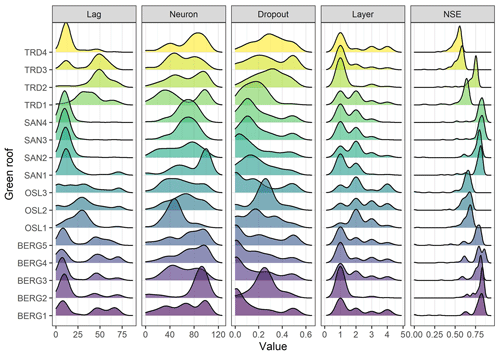Figure 3Empirical density distributions of the selected ANN hyperparameters by the Bayesian optimization algorithm and their associated performances in the validation datasets.

## 3.3 The conceptual retention model

The 16 roofs were modelled using a conceptual retention model (RM), which was developed and validated by . The RM model is intended to provide a robust tool that estimates green roof retention using simple water balance equations (Eqs. 9, 10 and 11).

$\begin{array}{}\text{(9)}& {R}_{t}=max\left(\mathrm{0},{P}_{t}-\left({S}_{\mathrm{max}}-{S}_{t}\right)-{\mathrm{AET}}_{t}\right),\text{(10)}& {S}_{t}=\mathrm{min}\mathrm{}\left({S}_{t-\mathrm{1}}+{P}_{t}-{\mathrm{AET}}_{t},\mathrm{0}\right),\text{(11)}& {\mathrm{AET}}_{t}={\mathrm{PET}}_{t}×\frac{{S}_{t-\mathrm{1}}}{{S}_{\mathrm{max}}},\end{array}$

Rt is the runoff from a green roof at time t, Pt is the precipitation at time t, Smax is the maximum storage available in a green roof and St is the water stored in a green roof at time t. In our study region, found the Oudin's model for ET to be the most accurate for their water balance model, and recommended the use of Oudin for cold climates. Hence, the potential evapotranspiration was computed using Oudin's model as follows (Eqs. 12):

$\begin{array}{}\text{(12)}& \begin{array}{rl}& \mathrm{PET}\left(\frac{\mathrm{mm}}{\mathrm{day}}\right)=\\ & \phantom{\rule{0.25em}{0ex}}\phantom{\rule{0.25em}{0ex}}\phantom{\rule{0.25em}{0ex}}\left\{\begin{array}{ll}\mathrm{0},& \text{if}\phantom{\rule{0.25em}{0ex}}{\mathrm{Ta}}_{\mathrm{mean}}\mathrm{\le }\mathrm{5}\phantom{\rule{0.125em}{0ex}}{}^{\circ }\mathrm{C};\\ \frac{\mathrm{Ra}}{\mathit{\lambda }\mathit{\rho }}×\mathrm{0.01}×\left({\mathrm{Ta}}_{\mathrm{mean}}+\mathrm{5}\right),& \text{if}\phantom{\rule{0.25em}{0ex}}{\mathrm{Ta}}_{\mathrm{mean}}>\mathrm{5}\phantom{\rule{0.125em}{0ex}}{}^{\circ }\mathrm{C}.\end{array}\right\\end{array}\end{array}$

Tamean is the daily mean temperature, Ra is extra-terrestrial radiation derived from Julian day and latitude (MJ m−2), $\frac{\mathrm{1}}{\mathit{\lambda }\mathit{\rho }}\approx \mathrm{0.408}$, λ is the latent heat of water (MJ kg−1) and ρ is the volumetric mass density of water (kg m−3).

The parameter Smax represents the maximum retention capacity of the green roof or the difference between the field capacity and the permanent wilting point of the green roof substrate . There exist standard laboratory tests to physically measure the substrate field capacity and the permanent wilting point . In this study, however, Smax values were estimated by assuming the field capacities of the roof layers from reported literature values as follow: vegetation mats were assumed to have 20 % of the total substrate depth as a field capacity , brick-based substrates were assumed to have 25 % of the total substrate depth as a field capacity and the drainage mats were assumed to have no permanent storage. The retention models with estimated Smax are referred to as uncalibrated retention models (RMuncalib).

To allow for fair comparison with the ML models, retention models with calibrated Smax values were used (RMcalib). For each roof, we ran the conceptual model by varying the value of Smax between 10 % to 50 % of the substrate total depth. Values of Smax that minimize the Volumetric error of the RM model were selected. The training periods in Table 2 were selected for calibration.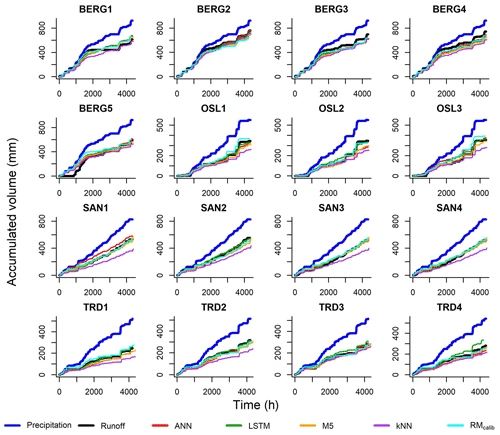Figure 5Cumulative precipitation, observed and simulated runoff of the green roofs.

## 3.4 ML model evaluation

Methods were evaluated based on the performance with the testing datasets. With respect to retention estimation, flow accumulation curves were plotted for the simulated runoff from ML models against the observed runoff and compared with the results from the conceptual retention model. In addition, the percentage bias (PBIAS) values (Eq. 13) were calculated for each simulation for comparison. To evaluate the performance of ML models in estimating the temporal variation in runoff, the simulated runoff from ML models were plotted against the observed values and the NSE (Eq. 14) values were determined. Values of NSE >0.5 were considered satisfactory . To evaluate the potential of using ML as a useful tool for planning and design purposes, ML models were transferred between the roofs unchanged. The transferred models simulated the testing periods of each roof, and NSE was used to evaluate the transferability performance. Moreover, a volumetric factor (vol) based on the PBIAS was determined by using Eq. (15) to assess transferability in terms of volume estimation. A vol value of 1 indicates a perfect runoff volume estimation and hence a perfect retention estimation, while a vol value of 0 indicates 100 % error in volume estimation. Additionally, we compared the performance of transferred ML models with the uncalibrated retention models.

$\begin{array}{}\text{(13)}& \mathrm{PBIAS}=\mathrm{100}×\frac{\sum {Q}_{\mathrm{obs}}-\sum {Q}_{\mathrm{sim}}}{\sum {Q}_{\mathrm{obs}}},\text{(14)}& \mathrm{NSE}=\mathrm{1}-\frac{\sum \left({Q}_{\mathrm{obs}}-{Q}_{\mathrm{sim}}{\right)}^{\mathrm{2}}}{\sum \left({Q}_{\mathrm{obs}}-\stackrel{\mathrm{‾}}{{Q}_{\mathrm{obs}}}{\right)}^{\mathrm{2}}},\text{(15)}& \mathrm{vol}=\mathrm{1}-\frac{|\mathrm{PBIAS}|}{\mathrm{100}}.\end{array}$
4 Results and discussion

## 4.1 Hyperparameter optimization (model tuning)

For LSTM and ANN models, the number of epochs was selected prior to the BO process by running an initial ANN model with 1000 epochs. After 30 epochs, the performance in the validation data did not improve further and started to decrease after 100 epochs while improving in the training dataset, which indicates overfitting. Therefore, 30 epochs were selected as an optimal value. Then, the BO algorithm was applied with 100 iterations for each ML model and for each roof. The selected hyperparameters of each iteration were stored. Figure 3 presents the empirical probability density distributions of the selected ANN hyperparameters by the BO and their associated performances in the validation datasets. The results can interpreted as that hyperparameters with high density values are located in regions that maximized the modelling performance in the validation datasets. The hyperparameters that generated the best results at the validation datasets were selected for each roof and each ML model, as presented in Table 4.

Based on Fig. 3, ANN with one hidden layer was found to be sufficient for most of the roofs in the study. Hence, deep ANN architectures, i.e. ANN models with many hidden layers, might not be required for this task. This has an important implication as deep ANN models are computationally expensive and prone to overfitting. Likewise, found that deep LSTM models are not required for predicting runoff at hourly time steps, while found a single-layer LSTM to perform better than an LSTM model with two layers for predicting daily water level depths in agricultural land.

Another interesting finding is the lag values which are varied between the cities. It can be noted that the lag values were smaller in Bergen and Sandnes compared to Trondheim and Oslo. To interpret this finding, rainfall events, with 6 h intra-event periods, were extracted from the three datasets at the four cities and compared, as shown in Fig. 4. Bergen roofs received events with higher amount and duration compared to Oslo and Trondheim roofs, whereas the antecedent dry weather periods (ADWPs) at Oslo and Trondheim are longer than BERG. Hence, due to the longer ADWP, a longer memory of the system is required to account for the wider range of possible initial saturation, compared to Bergen roofs.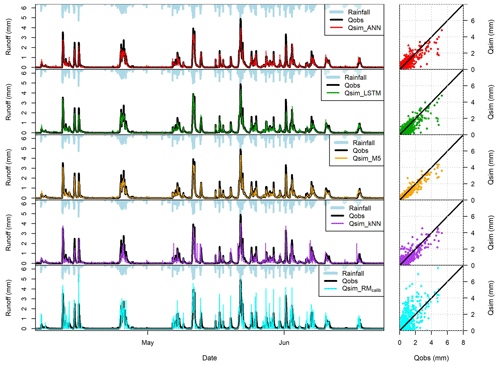Figure 6Performance of ML models on the testing period (BERG 2). The hydrographs were plotted for around 3-month period (2000 h), while the Q–Q plots were plotted for the entire testing period.

## 4.2 Model evaluations

### 4.2.1 Retention estimation

Machine learning models were built for all roofs based on the optimized hyperparameters, which were selected by the BO algorithm. Figure 5 illustrates the simulated and observed runoff cumulative curves together with the cumulative precipitation for each roof, and Table 5 shows the values of PBIAS and NSE of the models at the testing datasets. The results presented in Fig. 5 and Table 5 confirm that the ML models and the conceptual models can reproduce the observed runoff volume in most of the green roofs. By comparing the median values of the PBIAS on the testing periods, LSTM yielded only 0.15 % with a standard deviation of 8.61 %. Following LSTM, median values of 0.55 %, 1.50 % and 4.05 % were obtained by the RMcalib, ANN and RMuncalib models, respectively. The M5 models yielded simulation with a median PBIAS of 9.4 % while the kNN yielded the highest volumetric errors with a median PBIAS of 24.25 % with a standard deviation of 9.78 %. It can be noted that the conceptual retention models and ML models, except kNN, could produce results that are classified as acceptable modelling results regarding volumetric error (|PBIAS|< 25 %), as per .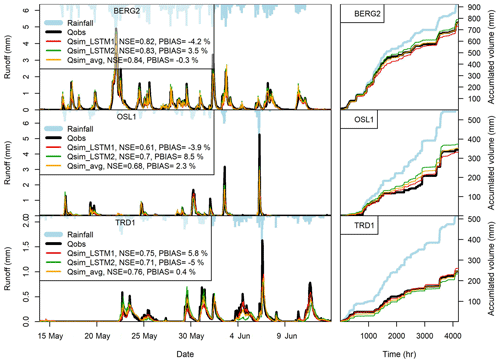Figure 8The performance of LSTM models in the testing datasets at BERG2, OSL1 and TRD1 roofs when using different data for model training. LSTM1 is trained using the testing period presented in Table 2, while LSTM2 is trained using the validation period presented in Table 2. Qsim-avg is the average of Qsim-LSTM1 and Qsim-LSTM2.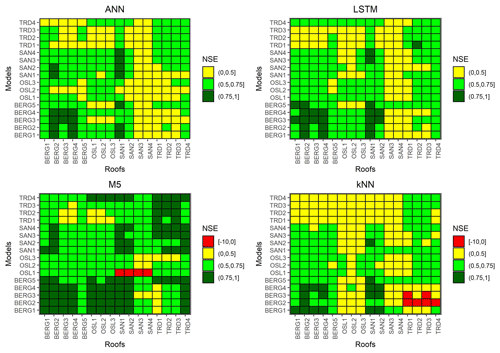Figure 9Transferability between the different roofs (NSE). Models on the y axis are used to simulate the measured green roofs on the x axis.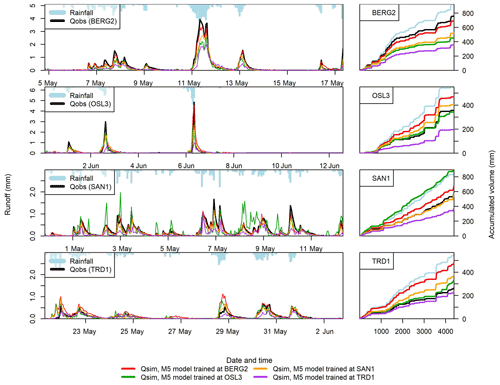Figure 10The performance of the transferred ML models at BERG2, OSL3, SAN1 and TRD1. The hydrographs were plotted for selected periods, while the cumulative plots were plotted for the entire testing period.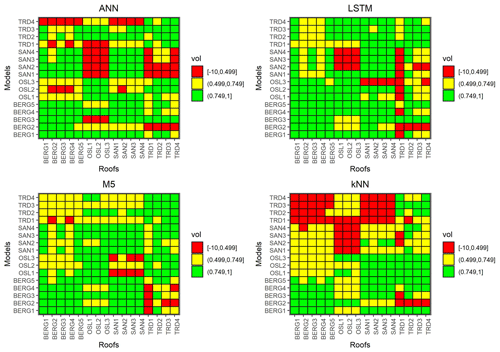Figure 11Transferability between the different roofs (vol). Models on the y axis are used to simulate the measured green roofs on the x axis.

### 4.2.2 Temporal variations in runoff

Table 5 presents the NSE values for training and validation periods for the ML models. Most ML models yielded satisfactory results in the testing periods (NSE > 0.5). M5 models produced results with highest NSE values, with a median value of 0.80. Both ANN and LSTM produced result with a median NSE values of 0.67. Figure 6 shows the observed and simulated hydrographs for BERG2 roof, which confirms the ability of the ML models to reproduce the observed runoff. In contrast, the conceptual models produced satisfactory results in only five roofs. We found the green roofs in our study to detain small- and medium-sized events for up to 2 h. The conceptual model failed to simulate these dynamics due to a lack of routing.

The performance of ML models varied between the different cities more than between the different configurations. , using the same data in this study, observed similar hydrological performance for the different configuration within the same city. However, it should be noted that the geometries of the roofs are identical at each city (Table 1). The performance of the ML methods can be explained based on this comparison between the cities’ rainfall characteristics (Fig. 4). For instance, the NSE values of the ML models are higher in Bergen roofs in comparisons to Oslo roofs. As mentioned earlier, Oslo roofs have a wider range of possible initial saturations. Therefore, 1 year of training data might not be enough to cover this wide range of runoff possibilities. On the other hand, Bergen roofs received more frequent and intense precipitation events resulting in a small range of possibilities of initial saturation that could be covered using 1 year only. The kNN method produced lower NSE values compared to the other ML models. This was attributed to the relatively small training data used in this study as kNN estimates the performance depending on the similarity to the previous time steps.

LSTM maintains a state value between consecutive time steps which makes it more suitable for modelling green roofs where initial saturation plays an important role in green roof runoff generation process. A comparison was made between ANN and LSTM at TRD1 (Fig. 7) to demonstrate the potential of LSTM. ANN was found to produce runoff when no precipitation occurred, unlike LSTM. Moreover, LSTM could simulate the flow peaks more accurately than ANN. Likewise, found LSTM simulations to be smoother than a normal recurrent neural network and to be better in accounting for the storage capacity (including snow accumulation) of a natural catchment

### 4.2.3 Effect of training data and ensemble modelling

The performance of ML models when using different data for model training was evaluated. For each roof, two ML models were built; one by using the training datasets in Table 2 for model training and one by using the validation datasets in Table 2 for model training. Sandnes roofs were excluded from this analysis due the missing data in one 2015, as discussed earlier. Figure 8 demonstrates the performance of LSTM models at BERG2, OSL1 and TRD1 roofs when using different data for model training. The performances of the two LSTM models (LSTM1 and LSTM2) were quite similar, as presented in Fig. 8. One idea that could improve the estimates of the ML models is to combine the simulations from several ML models that are build from different datasets (ensemble modelling). As shown in Fig. 8, the average simulations of two LSTM models yielded better simulation for the testing dataset (lower PBIAS and slightly higher NSE values) in comparison to the estimate of each of the two LSTM models. However, this is only true when the two LSTM models have positive and negative biases. Nevertheless, we found few green roofs where the two LSTM models from the 2 years resulted in biases of the same direction (at BERG3 and OSL3 roofs).

### 4.2.4 Transferability

Models were transferred between the roofs unchanged to simulate the testing periods. Figure 9 presents the transferability performance measured by NSE. Some models could yield satisfactory results in different locations (NSE >0.5). For instance, M5 models that were trained using data from Bergen roofs could yield satisfactory performance in almost all cities. Figure 10 presents an example of transferred M5 models between BERG2, OSL3,SAN1 and TRD1 roofs. It can be noted that models that are trained in wetter cities, such as Bergen, overestimated the flows at cities with lower precipitation, such as Trondheim. Figure 11 presents the transferability performance with respect to retention estimation measured by vol. Transferred ML model could simulate result with acceptable accuracy (vol >0.75) between Trondheim and Oslo cities and between Sandnes and Bergen cities with some exceptions. This can be somewhat attributed to the similarity in climatic conditions between the cities (Fig. 4). However, the uncalibrated conceptual models in this study could produce better volume estimates than the transferred ML models in most cases. This implies that using the conceptual model with literature estimates of the Smax parameter is preferable over the transferred ML to estimate the annual retention for new roofs.

## 4.3 Machine learning potentials for green roof hydrological modelling

The present paper has demonstrated that well-trained ML models can be applied to estimate retention process (rainfall losses) in a range of different green roof systems. The predictions are comparable in accuracy to a conceptual water balance model based on losses due to evapotranspiration. Additionally, well-trained ML models showed more accurate predictions of runoff hydrographs than the conceptual water balance model, which is encouraging for detention modelling. Moreover, aggregating the simulations of many ML models (ensemble modelling) appears to improve the prediction and can be investigated in future studies. Detention modelling is required to estimate the lag and attenuation of runoff associated with any rainfall that is not retained. In practice, many modelling frameworks rely on calibrated reservoir routing models to estimate the cumulative detention effects of multiple interacting component layers, and few (if any) convincing validation cases for a complete detention modelling framework have been presented. It would therefore be very valuable to explore whether the ML models, when trained on higher temporal resolution datasets, have the capability to capture these complex detention effects better than the alternative black-box approaches.

5 Conclusions

Four machine learning models, commonly used in runoff modelling studies, were applied to simulate runoff from 16 green roofs located in four Norwegian cities with different climatic conditions. We further investigated the potential of using ML models to estimate performance of new roofs where runoff data are not available for model training. This was done by means of transferring ML models between the roofs in the study. Our results confirm the ability of well-trained ML models to estimate green roof retention and the temporal runoff dynamics. The estimates of the annual retention were comparable to a proven conceptual model. Despite the 1 h time step, the ML models provided accurate simulations of runoff dynamics, i.e. discharge hydrographs (NSE values higher than 0.5 in most cases), which is encouraging for detention modelling. The LSTM demonstrated better modelling performance by maintaining a state value between consecutive time step, which makes it more appropriate for simulating runoff of green roofs. In future studies, shorter time steps will be applied to estimate detention metrics.

Some transferred ML models could give acceptable model performance (NSE >0.5, |PBIAS|<25 %) in different locations. However, we recommend using the conceptual model with literature values of the Smax parameter to estimate the annual retention of new roofs over the transferred model as it give accurate volume estimations.

Code availability

The code is available on reasonable request from the corresponding author.

Data availability

The datasets generated during and/or analysed during the current study are available from the corresponding author on reasonable request.

Author contributions

EMHA was responsible of the machine learning models, including the selection of the four models, hyperparameter tuning and models training. VS proposed the comparison with the conceptual retention model. VP assisted EMHA during ML modelling and the conceptual model application. KA and TMM supervised each step of the research from the start. EMHA wrote the first manuscript which was revised many times by all co-authors

Competing interests

The authors declare that they have no conflict of interest.

Disclaimer

Publisher’s note: Copernicus Publications remains neutral with regard to jurisdictional claims in published maps and institutional affiliations.

Acknowledgements

The authors would like to acknowledge the constructive comments from the reviewers and the editor.

Financial support

This research has been supported by the Norges Forskningsråd (grant no. SFI Klima2050 (237859/030)) (http://www.klima2050.no/).

Review statement

This paper was edited by Christa Kelleher and reviewed by three anonymous referees.

References

Allaire J. J. and Cholle, F.: keras: R Interface to 'Keras', R package version 2.2.5.0, available at: https://CRAN.R-project.org/package=keras (last access: 10 November 2021), 2019. a

Allen, R. G., Pereira, L. S., Raes, D., Smith, M.: Crop evapotranspiration-Guidelines for computing crop water requirements-FAO Irrigation and drainage paper 56, Fao, Rome, 300, D05109, 1998. a

Almorox, J., Quej, V. H., and Martí, P.: Global performance ranking of temperature-based approaches for evapotranspiration estimation considering Köppen climate classes, J. Hydrol., 528, 514–522, 2015. a

Ayzel, G.: Does deep learning advance hourly runoff predictions, in: Proceedings of the V International Conference Information Technologies and High-Performance Computing (ITHPC-2019), Khabarovsk, Russia, CEUR Workshop Proceedings (CEUR-WS.org), 16–19, 2019. a, b, c, d

Bengtsson, L., Grahn, L., and Olsson, J.: Hydrological function of a thin extensive green roof in southern Sweden, Hydrol. Res., 36, 259–268, 2005. a

Berndtsson, J. C.: Green roof performance towards management of runoff water quantity and quality: A review, Ecol. Eng., 36, 351–360, 2010. a

Berretta, C., Poë, S., and Stovin, V.: Reprint of “Moisture content behaviour in extensive green roofs during dry periods: The influence of vegetation and substrate characteristics”, J. Hydrol., 516, 37–49, 2014. a

Beygelzimer, A., Kakadet, S., Langford, J., Arya, S., Mount, D., Li, S., and Li, M. S.: Package “FNN”, available at: https://cran.r-project.org/web/packages/FNN/index.html, last access: 10 November 2021. a

Bhattacharya, B. and Solomatine, D. P.: Neural networks and M5 model trees in modelling water level–discharge relationship, Neurocomputing, 63, 381–396, 2005. a

Bouzouidja, R., Séré, G., Claverie, R., Ouvrard, S., Nuttens, L., and Lacroix, D.: Green roof aging: Quantifying the impact of substrate evolution on hydraulic performances at the lab-scale, J. Hydrol., 564, 416–423, 2018. a

Breuning, J. and Yanders, A.: FLL guidelines for the planning, construction and maintenance of green roofing, Green Roof Service LLC Baltimore, MD, USA, 2008. a

Brunetti, G., Šimůnek, J., and Piro, P.: A comprehensive analysis of the variably saturated hydraulic behavior of a green roof in a mediterranean climate, Vadose Zone J., 15, 1–17, 2016. a, b

Carson, T., Marasco, D., Culligan, P., and McGillis, W.: Hydrological performance of extensive green roofs in New York City: observations and multi-year modeling of three full-scale systems, Environ. Res. Lett., 8, 024036, https://doi.org/10.1088/1748-9326/8/2/024036, 2013. a

Cipolla, S. S., Maglionico, M., and Stojkov, I.: A long-term hydrological modelling of an extensive green roof by means of SWMM, Ecol. Eng., 95, 876–887, 2016. a

Daniel, T.: Neural networks – Applications in hydrology and water resources engineering, Proc., Int. Hydrology and Water Resources Symp., Vol. 3, 797–802, National Conference Publication 91/22, Institute of Engineers, Perth, Australia, 1991. a

DHI: MIKE URBAN Collection System. Modelling of Storm Water Drainage Networks and Sewer Collection Systems. User Guide, Danish Hydraulic Institute (DHI), Hørsholm, Denmark, 2017. a

Dunnett, N. and Kingsbury, N.: Planting Green Roofs and Living Walls, Timber Press, Cambridge, 2004. a

Fassman, E. and Simcock, R.: Moisture measurements as performance criteria for extensive living roof substrates, J. Environ. Eng., 138, 841–851, 2012. a

Fassman-Beck, E., Voyde, E., Simcock, R., and Hong, Y. S.: 4 Living roofs in 3 locations: Does configuration affect runoff mitigation?, J. Hydrol., 490, 11–20, 2013. a

Gharaei-Manesh, S., Fathzadeh, A., and Taghizadeh-Mehrjardi, R.: Comparison of artificial neural network and decision tree models in estimating spatial distribution of snow depth in a semi-arid region of Iran, Cold Reg. Sci. Technol., 122, 26–35, 2016. a

Goyal, M. K., Ojha, C., Singh, R., Swamee, P., Nema, R.: Application of ANN, fuzzy logic and decision tree algorithms for the development of reservoir operating rules, Water Resour. Manag., 27, 911–925, 2013a. a

Goyal, M. K., Ojha, C., Singh, R., Swamee, P.: Application of artificial neural network, fuzzy logic and decision tree algorithms for modelling of streamflow at Kasol in India, Water Sci. Technol., 68, 2521–2526, 2013b. a

Hernes, R. R., Gragne, A. S., Abdalla, E. M., Braskerud, B. C., Alfredsen, K., and Muthanna, T. M.: Assessing the effects of four SUDS scenarios on combined sewer overflows in Oslo, Norway: evaluating the low-impact development module of the Mike Urban model, Hydrol. Res., 51, 1437–1454, 2020. a

Hochreiter, S. and Schmidhuber, J.: Long short-term memory, Neural Comput., 9, 1735–1780, 1997. a

Hsu, K.-l., Gupta, H. V., and Sorooshian, S.: Artificial neural network modeling of the rainfall-runoff process, Water Resour. Res., 31, 2517–2530, 1995. a

Hu, C., Wu, Q., Li, H., Jian, S., Li, N., and Lou, Z.: Deep learning with a long short-term memory networks approach for rainfall-runoff simulation, Water, 10, 1543, https://doi.org/10.3390/w10111543, 2018. a

Jahanfar, A., Drake, J., Sleep, B., and Gharabaghi, B.: A modified FAO evapotranspiration model for refined water budget analysis for Green Roof systems, Ecol. Eng., 119, 45–53, 2018. a

Javan, K., Lialestani, M. R. F. H., and Nejadhossein, M.: A comparison of ANN and HSPF models for runoff simulation in Gharehsoo River watershed, Iran, Modeling Earth Systems and Environment, 1, 1–13, 2015. a

Johannessen, B. G., Hanslin, H. M., and Muthanna, T. M.: Green roof performance potential in cold and wet regions, Ecol. Eng., 106, 436–447, 2017. a

Johannessen, B. G., Muthanna, T. M., and Braskerud, B. C.: Detention and retention behavior of four extensive green roofs in three nordic climate zones, Water, 10, 671, https://doi.org/10.3390/w10060671, 2018. a, b, c, d, e, f

Johannessen, B. G., Hamouz, V., Gragne, A. S., and Muthanna, T. M.: The transferability of SWMM model parameters between green roofs with similar build-up, J. Hydrol., 569, 816–828, 2019. a

Karlsson, M. and Yakowitz, S.: Nearest-neighbor methods for nonparametric rainfall-runoff forecasting, Water Resour. Res., 23, 1300–1308, 1987. a

Kottek, M., Grieser, J., Beck, C., Rudolf, B., and Rubel, F.: World map of the Köppen-Geiger climate classification updated, Meteorol. Z., 15, 259–263, https://doi.org/10.1127/0941-2948/2006/0130, 2006. a

Kratzert, F., Klotz, D., Brenner, C., Schulz, K., and Herrnegger, M.: Rainfall–runoff modelling using Long Short-Term Memory (LSTM) networks, Hydrol. Earth Syst. Sci., 22, 6005–6022, https://doi.org/10.5194/hess-22-6005-2018, 2018. a, b, c, d

Kratzert, F., Klotz, D., Shalev, G., Klambauer, G., Hochreiter, S., and Nearing, G.: Towards learning universal, regional, and local hydrological behaviors via machine learning applied to large-sample datasets, Hydrol. Earth Syst. Sci., 23, 5089–5110, https://doi.org/10.5194/hess-23-5089-2019, 2019. a

Krebs, G., Kuoppamäki, K., Kokkonen, T., and Koivusalo, H.: Simulation of green roof test bed runoff, Hydrol. Process., 30, 250–262, 2016. a

Kuhn, M., Weston, S., Keefer, C., and Coulter, N.: Cubist models for regression, R package Vignette R package version 0.0, 18, available at: https://cran.r-project.org/web/packages/Cubist/index.html (last access: 10 November 2021), 2012. a

Li, S., Kazemi, H., and Rockaway, T. D.: Performance assessment of stormwater GI practices using artificial neural networks, Sci. Total Environ., 651, 2811–2819, 2019. a, b

Li, Y. and Babcock Jr., R. W.: Modeling hydrologic performance of a green roof system with HYDRUS-2D, J. Environ. Eng., 141, 04015036, https://doi.org/10.1061/(ASCE)EE.1943-7870.0000976, 2015. a

Liu, R. and Fassman-Beck, E.: Hydrologic response of engineered media in living roofs and bioretention to large rainfalls: experiments and modeling, Hydrol. Proc., 31, 556–572, 2017. a, b

Liu, X. and Chui, T. F. M.: Evaluation of green roof performance in mitigating the impact of extreme storms, Water, 11, 815, https://doi.org/10.3390/w11040815, 2019. a

Modaresi, F., Araghinejad, S., and Ebrahimi, K.: A comparative assessment of artificial neural network, generalized regression neural network, least-square support vector regression, and K-nearest neighbor regression for monthly streamflow forecasting in linear and nonlinear conditions, Water Resour. Manage., 32, 243–258, 2018. a

Moriasi, D. N., Arnold, J. G., Van Liew, M. W., Bingner, R. L., Harmel, R. D., and Veith, T. L.: Model evaluation guidelines for systematic quantification of accuracy in watershed simulations, T. ASABE, 50, 885–900, 2007. a, b, c

Multiphysics, C.: User Guide Version 4.4, COMSOL Multiphysics, Stockholm, Sweden, 2013. a

Palla, A., Gnecco, I., and Lanza, L. G.: Unsaturated 2D modelling of subsurface water flow in the coarse-grained porous matrix of a green roof, J. Hydrol., 379, 193–204, 2009. a

Palla, A., Gnecco, I., and Lanza, L.: Compared performance of a conceptual and a mechanistic hydrologic models of a green roof, Hydrol. Proc., 26, 73–84, 2012. a, b

Peng, Z. and Stovin, V.: Independent validation of the SWMM green roof module, J. Hydrol. Eng., 22, 04017037, https://doi.org/10.1061/(ASCE)HE.1943-5584.0001558, 2017. a

Peng, Z., Smith, C., and Stovin, V.: Internal fluctuations in green roof substrate moisture content during storm events: Monitored data and model simulations, J. Hydrol., 573, 872–884, 2019. a, b, c

Quinlan, J. R.: Combining instance-based and model-based learning, in: Proceedings of the tenth international conference on machine learning, 236–243, Amherst, Massachusetts, 27–29 June 1993. a

Quinlan, J. R.: Learning with continuous classes, in: 5th Australian joint conference on artificial intelligence, vol. 92, 343–348, World Scientific, 1992. a

Radfar, A. and Rockaway, T. D.: Captured runoff prediction model by permeable pavements using artificial neural networks, J. Infrastruct. Syst., 22, 04016007, https://doi.org/10.1061/(ASCE)IS.1943-555X.0000284, 2016. a, b

Rezaei, F., Jarrett, A., Berghage, R., and Beattie, D.: Evapotranspiration rates from extensive green roof plant species, in: 2005 ASAE Annual Meeting, p. 1, American Society of Agricultural and Biological Engineers, https://doi.org/10.13031/2013.18942, 2005. a

Rosa, D. J., Clausen, J. C., and Dietz, M. E.: Calibration and verification of SWMM for low impact development, J. Am. Water. Resour. As., 51, 746–757, 2015. a

Rossman, L. A.: Storm water management model user's manual, version 5.0., Cincinnati: National Risk Management Research Laboratory, Office of Research and Development, US Environmental Protection Agency, 2010. a

Rumelhart, D. E., Hinton, G. E., and Williams, R. J.: Learning representations by back-propagating errors, Nature, 323, 533–536, 1986. a

She, N. and Pang, J.: Physically based green roof model, J. Hydrol. Eng., 15, 458–464, 2010. a

Shen, C.: A transdisciplinary review of deep learning research and its relevance for water resources scientists, Water Resour. Res., 54, 8558–8593, 2018. a

Sherrard Jr., J. A. and Jacobs, J. M.: Vegetated roof water-balance model: experimental and model results, J. Hydrol. Eng., 17, 858–868, 2012. a

Shortridge, J. E., Guikema, S. D., and Zaitchik, B. F.: Machine learning methods for empirical streamflow simulation: a comparison of model accuracy, interpretability, and uncertainty in seasonal watersheds, Hydrol. Earth Syst. Sci., 20, 2611–2628, https://doi.org/10.5194/hess-20-2611-2016, 2016. a

Sims, A. W., Robinson, C. E., Smart, C. C., and O'Carroll, D. M.: Mechanisms controlling green roof peak flow rate attenuation, J. Hydrol., 577, 123972, https://doi.org/10.1016/j.jhydrol.2019.123972, 2019. a, b, c

Simunek, J., Vogel, T., and van Genuchten, M. T.: The SWMS_2D code for simulating water flow and solute transport in two-dimensional variably saturated media, US Salinity Laboratory, Agricultural Research Service, US Department of Agriculture, 1994. a

Simunek, J., Van Genuchten, M. T., and Sejna, M.: The HYDRUS-1D software package for simulating the one-dimensional movement of water, heat, and multiple solutes in variably-saturated media, University of California-Riverside Research Reports, 3, 1–240, Riverside, California, 2005. a

Snoek, J., Larochelle, H., and Adams, R. P.: Practical bayesian optimization of machine learning algorithms, Adv. Neur. In., 25, arXiv [preprint], arXiv:1206.2944, 2012. a, b, c

Solomatine, D. P. and Dulal, K. N.: Model trees as an alternative to neural networks in rainfall–runoff modelling, Hydrol. Sci. J., 48, 399–411, 2003. a, b, c, d

Soulis, K. X., Valiantzas, J. D., Ntoulas, N., Kargas, G., and Nektarios, P. A.: Simulation of green roof runoff under different substrate depths and vegetation covers by coupling a simple conceptual and a physically based hydrological model, J. Environ. Manage., 200, 434–445, 2017. a

Srivastava, N., Hinton, G., Krizhevsky, A., Sutskever, I., and Salakhutdinov, R.: Dropout: a simple way to prevent neural networks from overfitting, J. Mach. Learn. Res., 15, 1929–1958, 2014.  a

Stovin, V.: The potential of green roofs to manage urban stormwater, Water Environ. J., 24, 192–199, 2010. a

Stovin, V., Poë, S., and Berretta, C.: A modelling study of long term green roof retention performance, J. Environ. Manage., 131, 206–215, 2013. a, b, c, d, e

Tokar, A. S. and Johnson, P. A.: Rainfall-runoff modeling using artificial neural networks, J. Hydrol. Eng., 4, 232–239, 1999. a

Tsang, S. and Jim, C. Y.: Applying artificial intelligence modeling to optimize green roof irrigation, Energ. Buildings, 127, 360–369, 2016. a, b

Vesuviano, G. and Stovin, V.: A generic hydrological model for a green roof drainage layer, Water Sci. Technol., 68, 769–775, 2013. a

Vesuviano, G., Sonnenwald, F., and Stovin, V.: A two-stage storage routing model for green roof runoff detention, Water Sci. Technol., 69, 1191–1197, 2014. a

Wilson, S.: ParBayesianOptimization: Parallel Bayesian Optimization of Hyperparameters, available at: https://CRAN.R-project.org/package=ParBayesianOptimization (last access: 10 November 2021), r package version 1.2.4, 2021. a

Worland, S. C., Farmer, W. H., and Kiang, J. E.: Improving predictions of hydrological low-flow indices in ungaged basins using machine learning, Environ. Modell. Softw., 101, 169–182, 2018. a, b, c

Wu, C., Chau, K. W., and Li, Y. S.: Predicting monthly streamflow using data-driven models coupled with data-preprocessing techniques, Water Resour. Res., 45, 2009. a

Yilmaz, A. G. and Muttil, N.: Runoff estimation by machine learning methods and application to the Euphrates Basin in Turkey, J. Hydrol. Eng., 19, 1015–1025, 2014. a

Yio, M. H., Stovin, V., Werdin, J., and Vesuviano, G.: Experimental analysis of green roof substrate detention characteristics, Water Sci. Technol., 68, 1477–1486, 2013. a

Young, C.-C., Liu, W.-C., and Wu, M.-C.: A physically based and machine learning hybrid approach for accurate rainfall-runoff modeling during extreme typhoon events, Appl. Soft. Comput., 53, 205–216, 2017. a

Zhang, J., Zhu, Y., Zhang, X., Ye, M., and Yang, J.: Developing a Long Short-Term Memory (LSTM) based model for predicting water table depth in agricultural areas, J. Hydrol., 561, 918–929, 2018. a